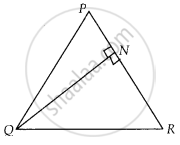# In a triangle ∆PQR, N is a point on PR such that QN ⊥ PR. If PN. NR = QN2, prove that ∠PQR = 90°. - Mathematics

Sum

In a triangle ∆PQR, N is a point on PR such that QN ⊥ PR. If PN. NR = QN2, prove that ∠PQR = 90°.

#### Solution

Given, ∆PQR, N is a point on PR, such that QN ⊥ PR and PN . NR = QN2

To prove: ∠PQR = 90°

Proof: We have, PN . NR = QNc

⇒ PN . NR = QN . QN

⇒ (PN)/(QN) = (QN)/(NR)  ......(i)

In ∆QNP and ∆RNQ,

(PN)/(QN) = (QN)/(NR)And ∠PNQ = ∠RNQ  ......[Each equal to 90°]

∴ ∆QNP ~ ∆RNQ   ......[By SAS similarity criterion]

Then, ∆QNP and ∆RNQ are equiangulars.

i.e., ∠PQN = ∠QRN

⇒ ∠RQN – ∠QPN

On adding both sides, we get

∠PQN + ∠RQN = ∠QRN + ∠QPN

⇒ ∠PQR = ∠QRN + ∠QPN   .........(ii)

We know that, sum of angles of a triangle is 180°

In ∆PQR, ∠PQR + ∠QPR + ∠QRP = 180°

⇒ ∠PQR + ∠QPN + ∠QRN = 180°

[∵∠QPR = ∠QPN and ∠QRP = ∠QRN]

⇒ ∠PQR + ∠PQR = 180°  .....[Using equation (ii)]

⇒ 2∠PQR = 180°

⇒ ∠PQR = 180^circ/2 = 90°

∴ ∠PQR = 90°

Hence proved.

Concept: Similarity of Triangles
Is there an error in this question or solution?

#### APPEARS IN

NCERT Mathematics Exemplar Class 10
Chapter 6 Triangles
Exercise 6.3 | Q 11 | Page 68
Share